# Pandas Styling: Exercises, Practice, Solution

[An editor is available at the bottom of the page to write and execute the scripts.]

Styling: This is a new feature and still under development. The styling is accomplished using CSS. You can write "style functions" that take scalars, DataFrames or Series, and return like-indexed DataFrames or Series with CSS "attribute: value" pairs for the values.

## Pandas Styling [15 exercises with solution]

1. Create a dataframe of ten rows, four columns with random values. Write a Pandas program to highlight the negative numbers red and positive numbers black. Go to the editor

Expected Output: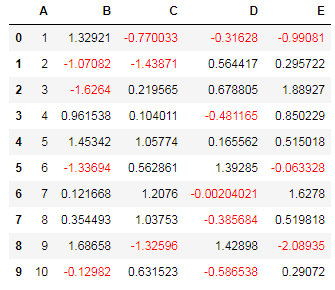Click me to see the sample solution

2. Create a dataframe of ten rows, four columns with random values. Convert some values to nan values. Write a Pandas program which will highlight the nan values. Go to the editor

Expected Output: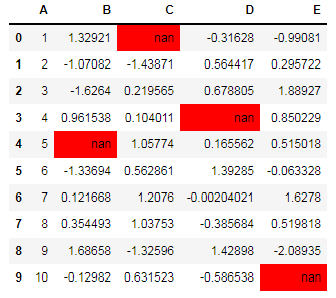Click me to see the sample solution

3. Create a dataframe of ten rows, four columns with random values. Write a Pandas program to highlight the maximum value in each column. Go to the editor

Expected Output: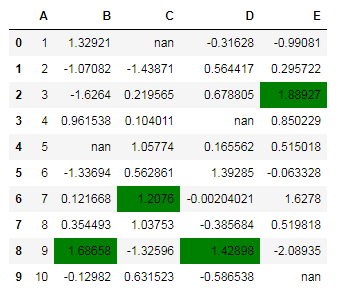Click me to see the sample solution

4. Create a dataframe of ten rows, four columns with random values. Write a Pandas program to highlight the minimum value in each column. Go to the editor

Expected Output: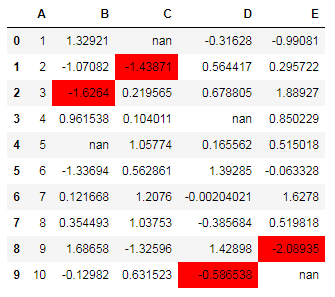Click me to see the sample solution

5. Create a dataframe of ten rows, four columns with random values. Write a Pandas program to highlight the maximum value in last two columns. Go to the editor

Expected Output: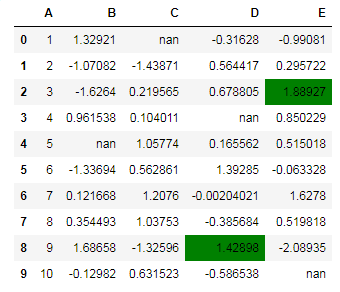Click me to see the sample solution

6. Create a dataframe of ten rows, four columns with random values. Write a Pandas program to set dataframe background Color black and font color yellow. Go to the editor

Expected Output: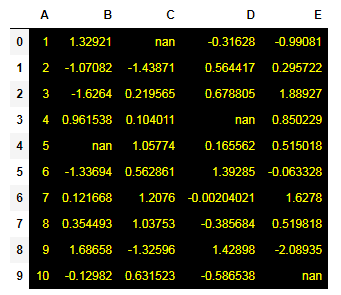Click me to see the sample solution

7. Create a dataframe of ten rows, four columns with random values. Write a Pandas program to highlight dataframe's specific columns. Go to the editor

Expected Output: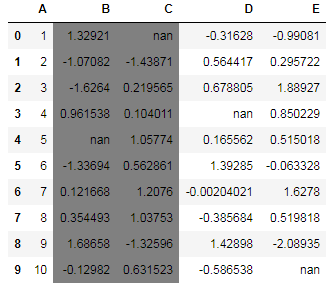Click me to see the sample solution

8. Create a dataframe of ten rows, four columns with random values. Write a Pandas program to highlight dataframe's specific columns with different colors. Go to the editor

Expected Output: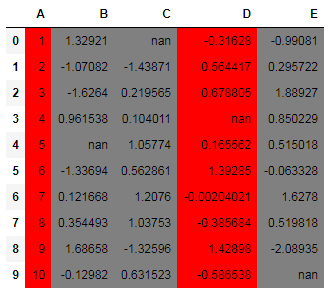Click me to see the sample solution

9. Create a dataframe of ten rows, four columns with random values. Write a Pandas program to display the dataframe in table style. Go to the editor

Expected Output: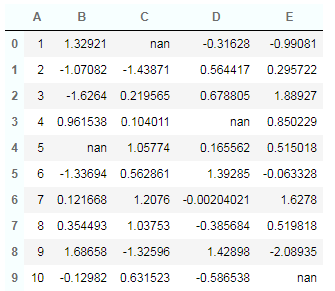Click me to see the sample solution

10. Create a dataframe of ten rows, four columns with random values. Write a Pandas program to highlight the entire row in Yellow where a specific column value is greater than 0.5. Go to the editor

Expected Output: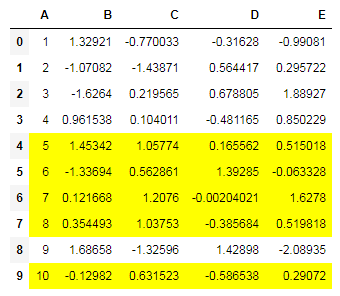Click me to see the sample solution

11. Create a dataframe of ten rows, four columns with random values. Write a Pandas program to display the dataframe in Heatmap style. Go to the editor

Expected Output: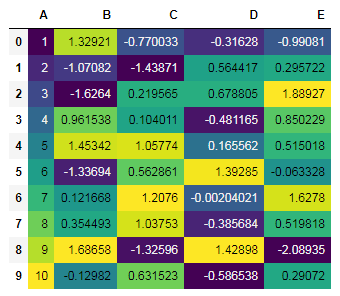Click me to see the sample solution

12. Create a dataframe of ten rows, four columns with random values. Write a Pandas program to make a gradient color mapping on a specified column. Go to the editor

Expected Output: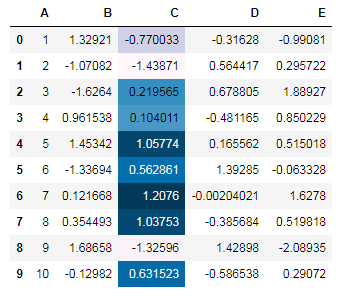Click me to see the sample solution

13. Create a dataframe of ten rows, four columns with random values. Write a Pandas program to make a gradient color on all the values of the said dataframe. Go to the editor

Expected Output: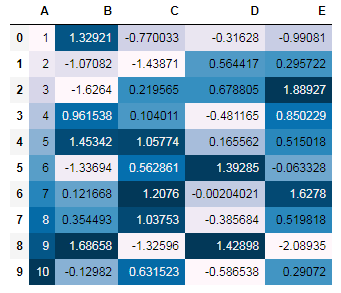Click me to see the sample solution

14. Create a dataframe of ten rows, four columns with random values. Write a Pandas program to display the dataframe in table style and border around the table and not around the rows. Go to the editor

Expected Output: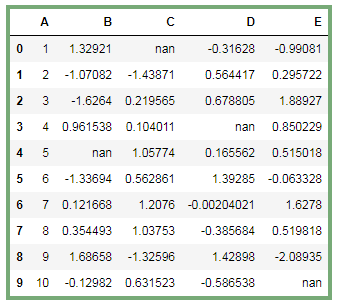Click me to see the sample solution

15. Create a dataframe of ten rows, four columns with random values. Write a Pandas program to display bar charts in dataframe on specified columns. Go to the editor

Expected Output: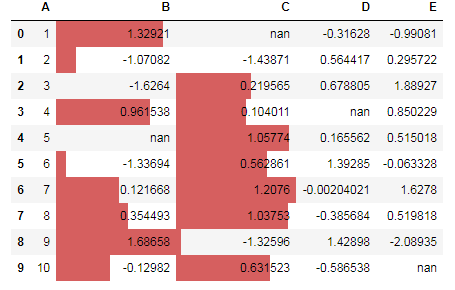Click me to see the sample solution

Python Code Editor:

More to Come !

Do not submit any solution of the above exercises at here, if you want to contribute go to the appropriate exercise page.

[ Want to contribute to Python Pandas exercises? Send your code (attached with a .zip file) to us at w3resource[at]yahoo[dot]com. Please avoid copyrighted materials.]

Test your Python skills with w3resource's quiz

﻿

## Python: Tips of the Day

Understanding slice notation:

It's pretty simple really:

```a[start:stop]  # items start through stop-1
a[start:]      # items start through the rest of the array
a[:stop]       # items from the beginning through stop-1
a[:]           # a copy of the whole array
```

There is also the step value, which can be used with any of the above:

```a[start:stop:step] # start through not past stop, by step
```

The key point to remember is that the :stop value represents the first value that is not in the selected slice. So, the difference between stop and start is the number of elements selected (if step is 1, the default).

The other feature is that start or stop may be a negative number, which means it counts from the end of the array instead of the beginning. So:

```a[-1]    # last item in the array
a[-2:]   # last two items in the array
a[:-2]   # everything except the last two items
```

Similarly, step may be a negative number:

```a[::-1]    # all items in the array, reversed
a[1::-1]   # the first two items, reversed
a[:-3:-1]  # the last two items, reversed
a[-3::-1]  # everything except the last two items, reversed
```

Python is kind to the programmer if there are fewer items than you ask for. For example, if you ask for a[:-2] and a only contains one element, you get an empty list instead of an error. Sometimes you would prefer the error, so you have to be aware that this may happen.

Relation to slice() object

The slicing operator [] is actually being used in the above code with a slice() object using the : notation (which is only valid within []), i.e.:

```a[start:stop:step]
```

is equivalent to:

```a[slice(start, stop, step)]
```

Slice objects also behave slightly differently depending on the number of arguments, similarly to range(), i.e. both slice(stop) and slice(start, stop[, step]) are supported. To skip specifying a given argument, one might use None, so that e.g. a[start:] is equivalent to a[slice(start, None)] or a[::-1] is equivalent to a[slice(None, None, -1)].

While the : -based notation is very helpful for simple slicing, the explicit use of slice() objects simplifies the programmatic generation of slicing.

Ref: https://bit.ly/2MHaTp7